### Home > CALC > Chapter 10 > Lesson 10.4.1 > Problem10-137

10-137.
1. For each of the geometric series state the common ratio “r” and determine the sum of the series. Homework Help ✎

1. 2.5 + 0.75 + 0.225 + 0.0675 + …

2.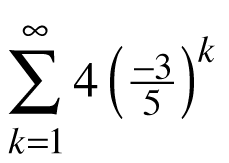3.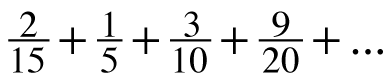4.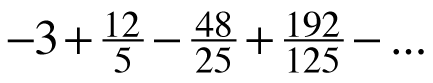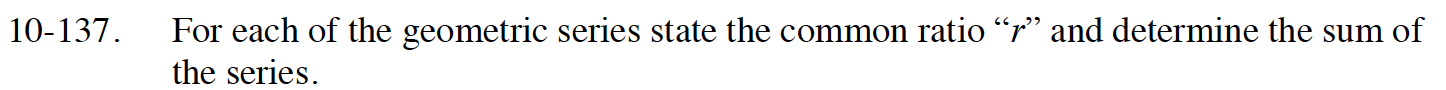$S=\frac{a}{1-r}$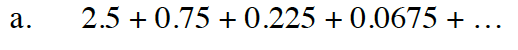$r=\frac{0.75}{2.5}$

$S=\frac{2.5}{1-0.75/2.5}$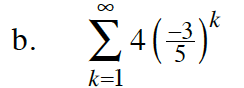$r=\frac{-3}{5}$

$a=4\Big(\frac{-3}{5}\Big)^1$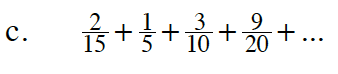$r=\frac{3}{2}$

What does this tell you about the sum of the series?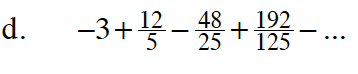$r=\frac{12/5}{-3}$